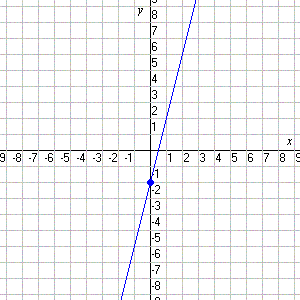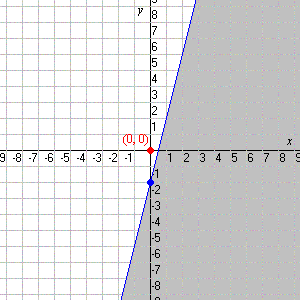# Graphing Linear Inequalities in Two Variables

To graph a linear inequality in two variables (say, $x$ and $y$ ), first get $y$ alone on one side. Then consider the related equation obtained by changing the inequality sign to an equals sign. The graph of this equation is a line.

If the inequality is strict ( $<$ or $>$ ), graph a dashed line. If the inequality is not strict ( $\le$ and $\ge$ ), graph a solid line.

Finally, pick one point not on the line ( $\left(0,0\right)$ is usually the easiest) and decide whether these coordinates satisfy the inequality or not. If they do, shade the half-plane containing that point. If they don't, shade the other half-plane.

Example:

Graph the inequality $y\le 4x-2$ .

This line is already in slope-intercept form , with $y$ alone on the left side. Its slope is $4$ and its $y$ -intercept is $-2$ . So it's straightforward to graph it. In this case, we make a solid line since we have a "less than or equal to" inequality.Now, substitute $x=0,y=0$ to decide whether $\left(0,0\right)$ satisfies the inequality.

$\begin{array}{l}0\stackrel{?}{\le }4\left(0\right)-2\\ 0\stackrel{?}{\le }-2\end{array}$

This is false. So, shade the half-plane which does not include the point $\left(0,0\right)$ .